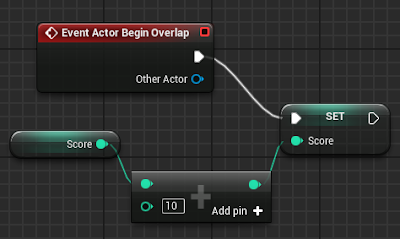## quarta-feira, 11 de maio de 2016

### Arithmetic Operators and Expressions

The arithmetic operators ( +, -, *, / ) can be used to create mathematical expressions in Blueprints. The image below shows a simple expression that add 10 points to the current player's score.Click to enlarge

The "+" operator receives two input values on the left and gives the operation result on the right. To use more than two input values just click on the "Add pin" option. The input values can be entered directly or can be obtained from variables.

As another example will be created an expression to calculate the "Attack Power" of a character. The expression uses the variables "Level" and "Strength" of the character. The expression is as follows:

Attack Power = ( Level x 3 ) + ( Strength x 2 – 20 )

To create an expression of this type in Blueprints is easier to identify the latest operations to be done and create the blueprint in the reverse order that resolves an expression, in other words, starting with the operation of lower precedence. In this example start with the "+" operator, whose input values ​​are the results of the small expressions that are inside the parentheses.Click to enlarge